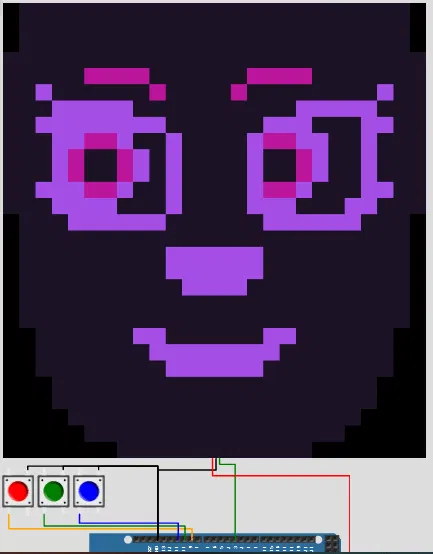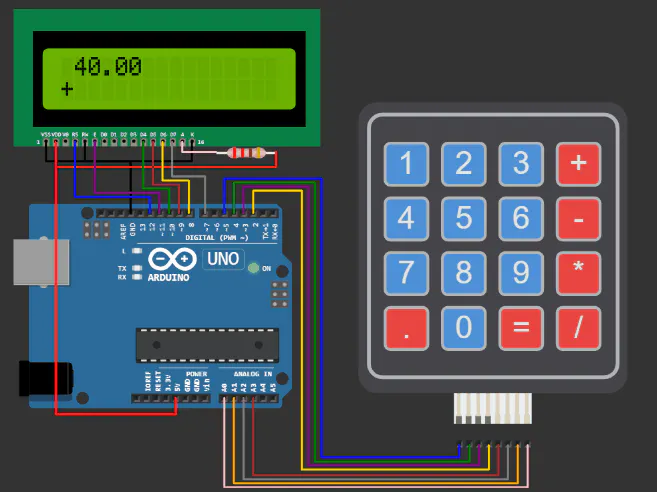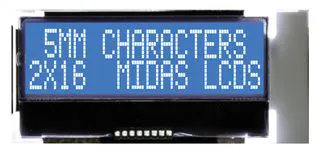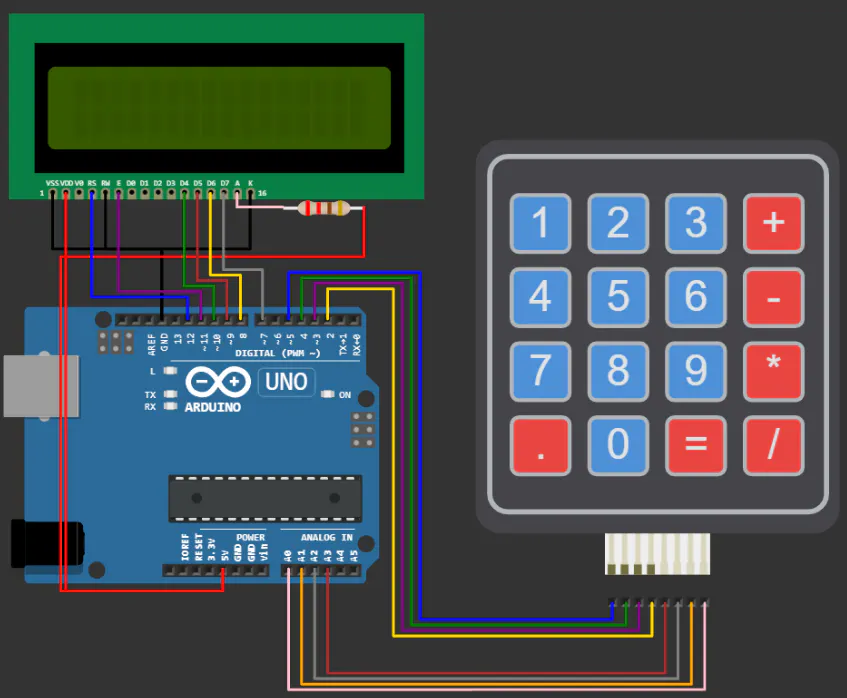# Build your calculator with Arduino UNO & LCD1602 display

This project provides you with a completely working code and connection diagram to set up your own calculator project using Arduino UNO

IntermediateFull instructions provided3## Things used in this project

### Hardware componentsArduino UNO
×1Alphanumeric LCD, 16 x 2
×1
×1

### Software apps and online services

 www.wokwi.com

## Schematics

### Connection diagram## Code

### Complete code - Arduino calculator

Arduino
```/**
Arduino Calculator

*/

#include <LiquidCrystal.h>
#include <Servo.h>

/* Display */
LiquidCrystal lcd(12, 11, 10, 9, 8, 7);

byte rowPins[KEYPAD_ROWS] = {5, 4, 3, 2};
byte colPins[KEYPAD_COLS] = {A3, A2, A1, A0};
{'1', '2', '3', '+'},
{'4', '5', '6', '-'},
{'7', '8', '9', '*'},
{'.', '0', '=', '/'}
};

uint64_t value = 0;

void showSpalshScreen() {
lcd.print("GoodArduinoCode");
lcd.setCursor(3, 1);
String message = "Calculator";
for (byte i = 0; i < message.length(); i++) {
lcd.print(message[i]);
delay(50);
}
delay(500);
}

void updateCursor() {
if (millis() / 250 % 2 == 0 ) {
lcd.cursor();
} else {
lcd.noCursor();
}
}

void setup() {
Serial.begin(115200);
lcd.begin(16, 2);

showSpalshScreen();
lcd.clear();
lcd.cursor();

lcd.setCursor(1, 0);
}

char operation = 0;
String memory = "";
String current = "";
uint64_t currentDecimal;
bool decimalPoint = false;

double calculate(char operation, double left, double right) {
switch (operation) {
case '+': return left + right;
case '-': return left - right;
case '*': return left * right;
case '/': return left / right;
}
}

void processInput(char key) {
if ('-' == key && current == "") {
current = "-";
lcd.print("-");
return;
}

switch (key) {
case '+':
case '-':
case '*':
case '/':
if (!operation) {
memory = current;
current = "";
}
operation = key;
lcd.setCursor(0, 1);
lcd.print(key);
lcd.setCursor(current.length() + 1, 1);
return;

case '=':
float leftNum = memory.toDouble();
float rightNum = current.toDouble();
memory = String(calculate(operation, leftNum, rightNum));
current = "";
lcd.clear();
lcd.setCursor(1, 0);
lcd.print(memory);
lcd.setCursor(0, 1);
lcd.print(operation);
return;

}

if ('.' == key && current.indexOf('.') >= 0) {
return;
}

if ('.' != key && current == "0") {
current = String(key);
} else if (key) {
current += String(key);
}

lcd.print(key);
}

void loop() {
updateCursor();

if (key) {
processInput(key);
}
}
```

## Credits

### puneeth kumar

7 projects • 1 follower
an Arduino enthusiast and an electronic hobbyist